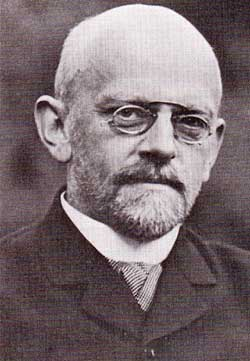A# Hilbert, David (1862–1943)David Hilbert was a German mathematician and one of the colossi of his subject in the 20th century. His most important discovery was of what is now called Hilbert space (see below). He was also a master of mathematical organization. During the early phase of his career, Hilbert reorganized number theory, crystallizing his conclusions in the classic book Der Zahlbericht (The Theory of Algebraic Number Fields, 1897). He then moved into geometry and performed a similar service by setting forth the first rigorous set of geometrical axioms in his Grundlagen der Geometrie (Foundations of Geometry, 1899). He invented a simple space-filling curve now known as the Hilbert curve and also proved Waring's conjecture.

At the Paris International Congress of 1900, Hilbert proposed 23 outstanding problems in mathematics to whose solutions he believed 20th-century mathematicians should devote themselves. These problems have come to be known as Hilbert's problems, and a number still remain unsolved today. Hilbert's mathematical philosophy is partly revealed by a couple remarks, one of which he made after learning that a student in his class had dropped the subject in order to become a poet. "Good," he said. "He did not have enough imagination to become a mathematician." Whether he really believed the second is open to question: "Mathematics is a game played according to certain simple rules with meaningless marks on paper."

Hilbert's dream was that it would be possible to prove or disprove all mathematical questions starting with only one set of well-defined rules and assumptions. In 1931 Kurt Gödel showed that this could not be achieved.

## Hilbert's axioms

In his Grundlagen der Geometries (the Foundations of Geometry), published in 1899, Hilbert developed 20 axioms intended to more adequately express Euclidean geometry. Euclid's original five axioms had long been viewed as incomplete and insufficiently rigorous. Hilbert's system begins with six primitive, undefined elements: three terms (point, straight line, plane) and three relations (betweenness – relating points; containment – relating points and straight lines, points and planes, and straight lines and planes; and congruence – relating line segments and angles).

Hilbert organised his axioms into five groups. The eight axioms of incidence refer to the occurrence and relation of points, lines, and planes in terms of "containment". The four axioms of order discuss them primarily in light of he concept of "betweenness". The six axioms of congruence refer to the equivalence relations of the basic terms, while the axiom of parallels defines the concept of a parallel line in Euclidean geometry. Finally, Hilbert concluded with two axioms of continuity, also known individually as Archimedes's axiom and linear completeness.

Hilbert's axioms evidenced an appreciation for clarity, organisation, and rigorous that shaped mathematics in the 20th century. Furthermore, they are indicative of what would become known as Hilbert's Program, the attempt to formulate mathematics on a system of axioms that is provably consistent. Ultimately, Hilbert's approach also promoted another monumental discovery:the proof by Kurt Gödel that no formal axiomatic system can substantiate its own completeness.

## Hilbert space

Hilbert space is a space of infinite dimensions in which distance is preserved by making the sum of squares of coordinates a convergent sequence; it is of crucial importance in the mathematical formulation of quantum mechanics.